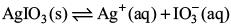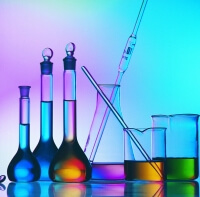Courses

Competition Level Test: Solutions Notes | Study Chemistry Class 12 - Class 12

Class 12: Competition Level Test: Solutions Notes | Study Chemistry Class 12 - Class 12

The document Competition Level Test: Solutions Notes | Study Chemistry Class 12 - Class 12 is a part of the Class 12 Course Chemistry Class 12.
All you need of Class 12 at this link: Class 12

Try yourself:Which one of the following aqueous solutions will exhibit highest boiling point?

Try yourself:6.02 x 1020 molecules of urea are present in 100 mL of its solution. The concentration of urea solution is
(Avogadro constant, NA  = 6.02 x 1023 mol–1)

Try yourself:Which of the following liquid pairs shows a positive deviation from Raoult’s law?

Try yourself:Which one of the following statements is false?

Try yourself:If α is the degree of dissociation of Na2SO4, the van’t Hoff factor used for the calculation of molecular mass is

Try yourself:Benzene and toluene form nearly ideal solutions. At 20°C, the vapour pressure of benzene is 75 Torr and that of toluene is 22 Torr. The partial vapour pressure of benzene at 20°C for a solution containing 78 g of benzene and 46 g toluene in Torr is

Try yourself:Equimolar solutions in the same solvent have

Try yourself:Among the following mixtures, dipole-dipole as the major interaction, is present in

Try yourself:18 g of glucose (C6H12O6) is added to 178.2 g of water. The vapour pressure of water for this aqueous solution at 100°C is

Try yourself:A 5.25% solution of a substance is isotonic with a 1.5% solution of urea (molar mass = 60 g mol–1) in the same solvent. If the densities of both the solutions are assumed to be equal to 1.0 g cm–3, molar mass of the substance will be

Try yourself:In a saturated solution of the sparingly soluble electrolyte AgIO3 (relative molecular mass = 283) the equilibrium which sets in isIf the solubility product constant Ksp of AgIO3 at a given temperature is 1.0 x 10–8 M, what is the mass of AgIO3 contained in 100 mL of its saturated solution?

Try yourself:A mixture of ethyl alcohol and propyl alcohol has a vapour pressure of 290 mmHg at 300 K. The vapour pressure of propyl alcohol is 200 mmHg. If the mole fraction of ethyl alcohol is 0.6, its vapour pressure (in mmHg) at the same temperature will be

Try yourself:At 80 °C, the vapour pressure of pure liquid A is 520 mmHg and that of pure liquid B is 1000 mmHg. If a solution of A and B boils at 80 °C and 1 atm pressure, the amount per cent of A in the mixture is

Try yourself:The vapour pressure of water at 20°C is 17.5 mmHg. If 18 g of glucose (C6H12O6) is added to 178.2 g of water at 20°C, the vapour of the resulting solution will be

Try yourself:Two liquids X and Y form an ideal solution. At 300 K, vapour pressure of the solution containing 1 mol of X and 3 mol of Y is 550 mmHg. At the same temperature, if 1 mol of Y is further added to this solution, vapour pressure of the solution increases by 10 mmHg. The vapour pressures (in mmHg) of X and Y in their pure states, respectively, will be

Try yourself:A binary liquid solution is prepared by mixing n-heptane and ethanol. Which one of the following statements is correct regarding the behaviour of the solution?

Try yourself: If sodium sulphate is considered to be completely dissociated into cations and anions in aqueous solution, the decrease in freezing point of water (ΔTf) when 0.01 mol of sodium sulphate is dissolved in 1 kg of water, (Kf = 1.86 K kg mol–1) is

Try yourself:On mixing, heptane and octane form an ideal solution. At 373 K, the vapour pressures of the two liquid components (heptane and octane) are 105 kPa and 45 kPa, respectively. Vapour pressure of the solution obtained by mixing 25.0 g of heptane (molar mass = 100 g mol–1) and 35.0 g of octane (molar mass of octane = 114 g mol–1) will be

Try yourself:Ethylene glycol is used as an antifreeze in a cold climate. Mass of ethylene glycol which should be added to 4 kg of water to prevent it from freezing at –6°C will be (Kf for water = 1.86 K kg mol–1 and molar mass of ethylene glycol = 62 g mol–1)

Try yourself:The degree of dissociation (α) of a weak electrolyte AxBy is related to van’t Hoff factor (i) by the expression

Try yourself:A 5.2 molal aqueous solution of methyl alcohol, CH3OH is supplied. What is the mole fraction of methyl alcohol in the solution?

Try yourself:A 5 % solution of cane sugar (molar mass: 342 g mol–1) is isotonic with 1% of a solution of an unknown solute. The molar mass of unknown solute in g/mol is

Try yourself:The molality of a urea solution in which 0.0100 g of urea, [(NH2)2CO], is added to 0.3000 dm3 of water at STP is

Try yourself:Kf for water is 1.86 K kg mol–1. If your automobile radiator holds 1.0 kg of water, how many grams of ethylene glycol (C2H6O2) must you add to get the freezing point of the solution lowered to – 2.8°C?

Try yourself:Consider separate solutions of 0.500 M C2H5OH(aq), 0.100 M Mg3(PO4)2 (aq), 0.250 M KBr (aq) and 0.125 M Na3PO4(aq) at 50°C. Which statement is true about these solutions, assuming all salts to be strong electrolytes?

The document Competition Level Test: Solutions Notes | Study Chemistry Class 12 - Class 12 is a part of the Class 12 Course Chemistry Class 12.
All you need of Class 12 at this link: Class 12Use Code STAYHOME200 and get INR 200 additional OFF Use Coupon CodeChemistry Class 12

105 videos|274 docs|259 tests

Track your progress, build streaks, highlight & save important lessons and more!

,

,

,

,

,

,

,

,

,

,

,

,

,

,

,

,

,

,

,

,

,

;